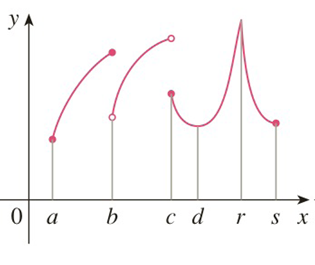Chapter 3.1, Problem 4E

Chapter
Section
Textbook Problem

# For each of the numbers a, b, c, d, r, and s, state whether the function whose graph is shown has an absolute maximum or minimum, a local maximum or minimum, or neither a maximum nor a minimum.To determine

To state:

Whether each of the numbers a, b, c, d, r, and s has an absolute maximum or minimum, a local maximum or minimum, or neither a maximum nor a minimum.

Explanation

1) Concept:

Use definitions and graph to find whether the given numberhas an absolute maximum or minimum, a local maximum or minimum, or neither a maximum nor a minimum.

2) Definitions:

i. Let c be a number in the domain D of a function f; then f(c) is the absolute maximum value of f on D if fcf(x) for all x in D

ii. Let c be a number in the domain D of a function f; then f(c) is the absolute minimum value of f on D if fcf(x) for all x in D.

iii. The number fc is a local maximum value of f if fcf(x) when x is near c.

iv. The number fc is a local minimum value of f if fcf(x) when x is near c.

3) Calculation:

In the given graph, at point a, the function has the smallest value, and at point r,  it has the greatest value.

Thus, (r, f(r)) is the highest point on the graph, and (a, f(a)) is the lowest point on the graph.

Therefore, by using the definition of absolute maximum and absolute minimum, absolute maximum is at point r, and absolute minimum is at point a.

Now consider the values of f(x)  near point b in a small open interval containing b

### Still sussing out bartleby?

Check out a sample textbook solution.

See a sample solution

#### The Solution to Your Study Problems

Bartleby provides explanations to thousands of textbook problems written by our experts, many with advanced degrees!

Get Started

#### Sketch a graph of each of the following functions.

Mathematical Applications for the Management, Life, and Social Sciences

#### Calculate y'. 22. y = sec(1 + x2)

Single Variable Calculus: Early Transcendentals

#### Simplify each expression. 22

Trigonometry (MindTap Course List)

#### Write the following numbers in word form. \$119.85

Contemporary Mathematics for Business & Consumers

#### limx0sin5x3x=. a) 1 b) 53 c) 35 d) 0

Study Guide for Stewart's Single Variable Calculus: Early Transcendentals, 8th